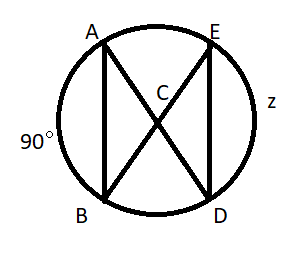Courses
Courses for Kids
Free study material
Free LIVE classes
MoreLIVE
Join Vedantu’s FREE Mastercalss

# In the circle shown below, AB = 3 and DE = 3. What is the value of z?Verified
$\because {\text{AB}} \cong {\text{DE}} \\ \therefore {\text{arc(AB) = arc(DE)}} \\ \Rightarrow {\text{ z = }}{90^ \circ } \\$
Hence, the required answer is z = ${90^ \circ }$